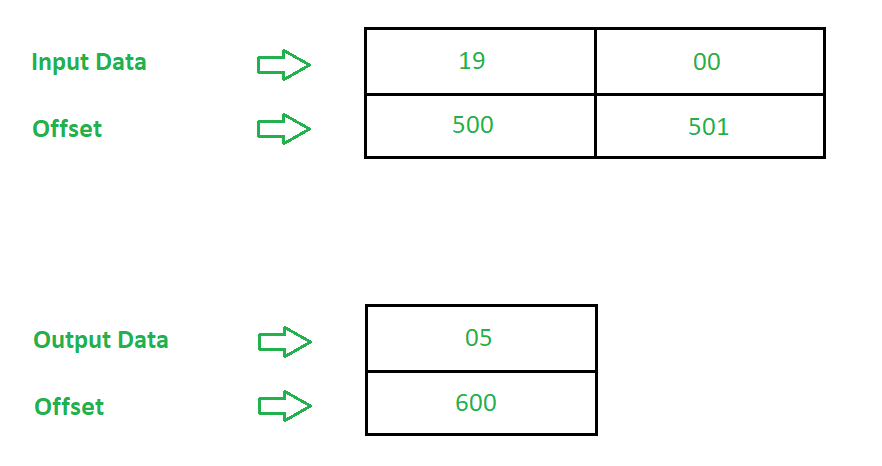# 8086 program to find Square Root of a number

Problem – Write an assembly language program in 8086 microprocessor to find square root of a number.

Example –Algorithm –

1. Move the input data in register AX
2. Move the data 0000 in CX and FFFF in BX
3. Add 0002 to the contents of BX
4. Increment the content of CX by 1
5. Subtract the contents of AX and BX
6. If Zero Flag(ZF) is not set go to step 3 else go to step 7
7. Store the data from CX to offset 600
8. Stop
9. Program –

OFFSET MNEMONICS COMMENT
0400 MOV AX,  AX <- 
0404 MOV CX, 0000 CX <- 0000
0407 MOV BX, FFFF BX <- FFFF
040A ADD BX, 02 BX = BX + 02
040E INC CX C = C + 1
040F SUB AX, BX AX = AX – BX
0413 MOV , CX  <- CX
0417 HLT Stop

Explanation –

1. M0V AX,  is used to move the data from offset 500 to register AX
2. MOV CX 0000 is used to move 0000 to register CX
3. MOV BX FFFF is used to move FFFF to register BX
5. INC CX is used to increment the content of CX by 1
6. SUB AX, BX is used to subtract contents of AX with BX